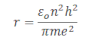# Bohr’s Atomic Model

Postulates of Bohr’s atomic model

Electrones revolve around the nucleus in certain permieteed orbit.
Angular momentum of the electron when it revolves arount the certain permitted orbit is equal to the integral multiple of h/2π
Mathematically, it can be expressed as: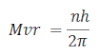Where n = 1 , 2, 3, ….
H = plank’s constant
M = mass of electron
V= velocity of electron
R = radius of orbit of electron

This principle is called a quantization principle.
When electron revolves around the nucleus in certain permitted orbit, it can have a transition from higher energy level to the lower energy level with the emission of radiation which corresponds to the difference in energy between any two energy levels.
The electrostatics force of attraction between the proton and the election is given by: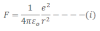If m and v be the mass and velocity of the electron in the orbit, then the centripetal force required by the electron to move in circular orbit of radius ‘r’ is given by: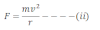Further, the electrostatic force of attraction between the election and the nucleus provides the necessary centripetal force.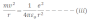According to Bohr’s postulate,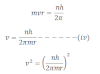Now, substituting the value of v2 in equation iii we get,